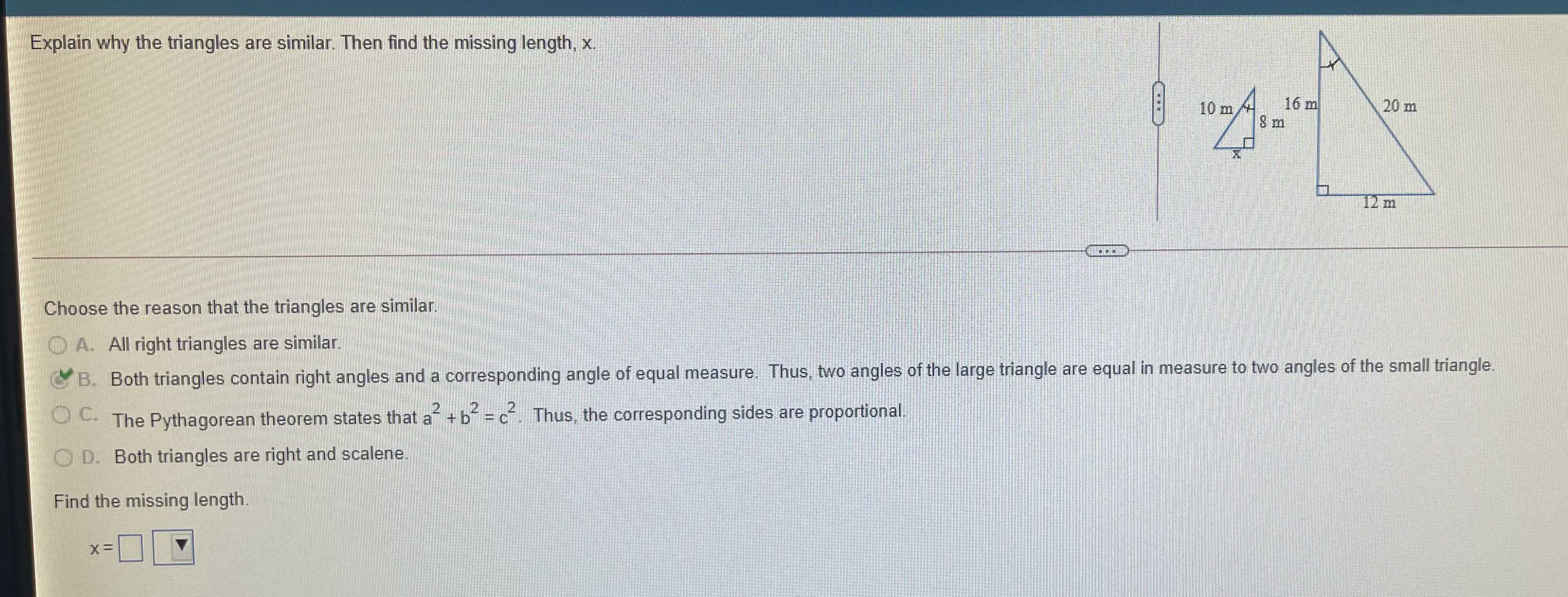### Still have math questions?Explain why the triangles are similar. Then find the missing length, $$x$$ . Choose the reason that the triangles are similar. A. All right triangles are similar. B. Both triangles contain right angles and a corresponding angle of equal measure. Thus, two angles of the large triangle are equal in measure to two angles of the small triangle. The Pythagorean theorem states that $$a ^ { 2 } + b ^ { 2 } = c ^ { 2 }$$ . Thus, the corresponding sides are proportional. Both triangles are right and scalene.